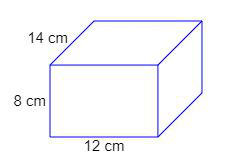# Volume of a cuboid calculator

* * =
Your browser does not support the HTML5 canvas tag.

### Volume calculators

This is a calculator that specifically calculates the volume of a cuboid, support metric and imperial units (inches, feet, yards, mm, cm or meter), and volume result can convert to different unit, with calculation formula and dynamic visual cube, it helps us to get answers and understand the results more easily.

### How to use this calculator ?

1. Input the numbers into blanks of length, width and height
2. Accept input decimals or fraction, eg. 2.6, 7.8, 4 1/2 or 3/5
3. Choose the unit of measure you are using (in, ft, yd, mm, cm, m)
4. Choose the unit of result you would like
5. It will automatically calculate the results and react interactively.
6. The result of the calculation will be rounded.

### How to calculate the volume of a cuboid

Cuboid is a solid box whose every surface is a rectangle of same area or different areas.
A cuboid will have a length, breadth and height.

Volume of a cuboid = (length × breadth × height) cubic units.

### Rectangle Volume Formula

Volume = length × width × height

### Calculation example

Find the volume of a cuboid of dimensions 14 cm × 12 cm × 8 cm.14 × 12 × 8 cubic cm.
= 1344 cubic cm.
Therefore, volume of cuboid = 1344 cubic cm.

If we want to convert units of volume into different units, we can convert the units of dimensions to same of volume first,
for example,
a cube has dimensions of 12.5 in, 14 in and 9.3 in.
What is it's volume in ft³?

12.5 in = 12.5 ÷ 12 ft = 1.042 ft
14 in = 14 ÷ 12 ft = 1.167 ft
9.3 in = 9.3 ÷ 12 ft = 0.775 ft
1.042 × 1.167 × 0.775 in = 0.94241085 ft³
after rounding, the volume is 0.94 in ft³

### Cuboid and Cube

Acuboidis a box-shaped object. It has six flat faces and all angles are right angles. And all of its faces are rectangles. It is also a prism because it has the same cross-section along a length. In fact it is a rectangular prism.

When all three lengths are equal it is called acube(or hexahedron) and each face is a square. A cube is still a prism and also a cuboid.